# Study of Eddy Current Characterization in Waste Particles

Study of Eddy Current Characterization in Waste Particles
Research Laboratory, Intelligent Control and Electrical Power Systems (ICEPS), Faculty of Electrical Engineering, Djilali Liabes University, Sidi Bel abbes 22000, Algeria

Applied Materials Laboratory, Faculty of Electrical Engineering, Djillali Liabès University, Sidi Bel abbes 22000, Algeria

Corresponding Author Email:
mimouna.oukli@univ-sba.dz
Page:
103-107
|
DOI:
https://doi.org/10.18280/ama_c.742-409
2 January 2019
|
Accepted:
7 September 2019
|
Published:
30 December 2019
| Citation

OPEN ACCESS

Abstract:

Non-ferrous metal waste particles from production falls, process industry scrap, and collected end-of-life products are used to make secondary (refined) metal, particles identification is paramount to facilitate their recycling. Eddy current testing is a widely used technique in non-destructive testing and evaluation of materials. The aim of the present study is the characterization optimization of particle waste by the eddy current method. Finite element 3D configurations are used. The particles thickness depends on the grinding machines. The air gap (lift off) between the control sensor and the waste can be adjusted. We performed a series of simulations by varying the value of the particle thickness, and the value of the lift off. The particle thickness has a significant effect on the sensor response. Also, the sensitivity of the sensor response increases with the decrease of the lift off value. Characterization of material particles depending on particle thickness and setting the value of lift off is realized by constructing an inverse model.

Keywords:

waste, COMSOL multiphysics, inverse model, optimization, eddy current

1. Introduction

The production capacity of materials is closely linked to natural resources and waste recycling opportunities. The accumulation of equipment and consumer goods is accompanied by a more localized waste material. This poses the problem of recycling materials . The first step in metal recycling is its separation from other materials. The source of the metal scrap is ground to have ferrous and non-ferrous metal particles . The waste characterization is essential to determine the most suitable and beneficial treatment method . The CF non-destructive testing method is one of the most used techniques for the inspection of conductive materials. This control process makes it possible to determine the characteristics of the part such as: electrical conductivity, magnetic permeability, size, coating, and to detect some anomalies in the metallic structure . The CF control is the process of creating induced current in a conductor placed below the control sensor which is traversed by a sinusoidal current (see Figure 1). Any local variation of the electrical (conductivity) or geometrical properties (dimensions, gap…) modifies the Z impedance at the terminals of the control sensor .

The characterization of the conductive materials particles by CF is achieved by the inversion of a direct model. This model is obtained by applying the least square method  on sensor-particle interaction signals, and simulation results are validated by experimental results . The aim of the present study is the particle waste characterization optimization by the eddy current method. Finite element 3D configurations are used. The thickness of the particles depends on the shearing procedure by the grinding machines. The air gap between the control sensor and the waste can be adjusted. We performed a series of simulations by varying the value of the particle thickness, and the value of the gap. The particle thickness has a significant effect on the sensor response. Also, the sensitivity of the sensor response increases with the decrease of the value of the gap. Materials characterization depending on the particle thickness and setting of the air gap value is achieved by constructing an inverse model.

## 1.jpg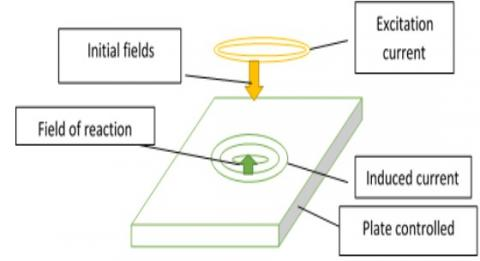Figure 1. The eddy current control principle

2. Using the COSMOL Multiphysics

COMSOL Multiphysics appeared to be best suited to the problem of CND-CF . Its particularity is to have a database of equations allowing to modelized different physical phenomena, as the problem dealt with electromagnetism. Advanced features make it possible to modify the specific computer. The CND-CF device to be studied is composed of an absolute pancake type sensor operating on a sample of particles of circular shape Figure 2.

Configurations are made in the electrical and magnetic field interface below the AC/DC module of COMSOL Multiphysics® Version 5.2a. The frequency used in the tests is low, therefore the CF control is applied in a quasi-static regime, i.e., displacement currents are neglected (Approximation of quasi-stationary ARQS regimes). The presence of a particle sample implies a 3D FEM resolution of the following equations for the magnetic vector potential A and electric scalar potentials V:

## 2.jpg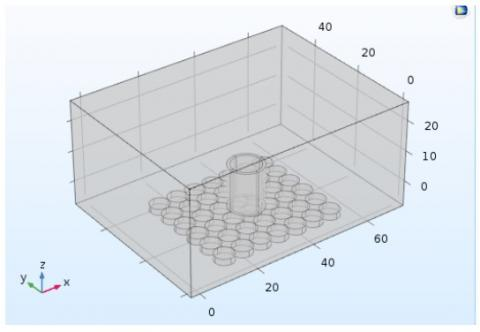Figure 2. Air box containing the control device

$-\nabla \cdot\left(\left(j \omega \sigma-\omega^{2} \varepsilon_{0}\right) A-\sigma v \times(\nabla \times A)+\left(\sigma+j \omega \varepsilon_{0}\right) \nabla V\right.$$\left.-\left(J_{e}+j \omega P\right)\right)=0 \left(j \omega \sigma-\omega^{2} \varepsilon_{0}\right) A+\nabla \times\left(\mu_{0}^{-1} \nabla \times A-M\right)-\sigma v \times(\nabla \times A)$$+\left(\sigma+j \omega \varepsilon_{0}\right) \nabla V-j \omega P=J_{e}$

where, ω is the circular angular frequency of excitation, σ is the material conductance, A is the magnetic potential vector, Je is the current density in a medium and B is the magnetic flux density, Figures 3 shows the magnetic flux density of the system coil-particles sample.

Once the values of the magnetic vector potential and the electric scalar potential are determined by the finite element method, the coil impedance that is our parameter of interest can be calculated. The impedance Z is composed of a real part R determined from the calculation of the losses by Joule effect in the conducting domain (waste), and of an imaginary part X calculated from the magnetic energy stored in the set of the system (sensor/waste). The expressions linking resistance to Joule loss and the magnetic energy reactance are given by the following relations :

$\left\{\begin{array}{c}R=\frac{1}{I^{2}} P_{J} \\ P_{J}=\frac{1}{2} \iiint_{\text {conducteur }} \frac{1}{\sigma}|\vec{J}|^{2} d v\end{array}\right.$  P   (1)

$\left\{\begin{array}{c}\mathrm{X}=\frac{2 \omega}{\mathrm{I}^{2}} \mathrm{W}_{\mathrm{m}} \\ \mathrm{W}_{\mathrm{m}}=\frac{1}{2} \iiint_{\mathrm{system}} \frac{1}{\mu}|\overrightarrow{\mathrm{B}}|^{2} \mathrm{d} v\end{array}\right.$  (2)

with $\vec{B}$ and $\mu$ respectively represent the magnetic induction and the magnetic permeability, $\vec{J}$ and $\sigma$ represent respectively the eddy current density and the electrical conductivity. In our work, the total impedance of the control sensor is calculated using the formula of electromagnetic energies and joules losses (1) and (2).

## 3.jpgFigure 3. Density of magnetic flux

3. Description of the CND-CF System to Study

Numerical simulation, helping us to make a different test configuration; flexibility, is not limited to the availability of samples. The characteristics of the simulation model are as follows:

1. The particles have a small size ranging from 4 to 10 mm ,
2. During the control test, eddy currents will be generated in the particle due to the interaction with the magnetic field of the coil, depending on the eddy current analysis induced in the metal particles, and according to the skin effect,
3. The shape of the currents induced in the particles is generated according to the shape and the maximum surface of the particle . The main diameters D of the chosen particles relating to the eddy current process are established by determining the size of the largest circle (Eddy current) see Figure 4.

## 4.jpg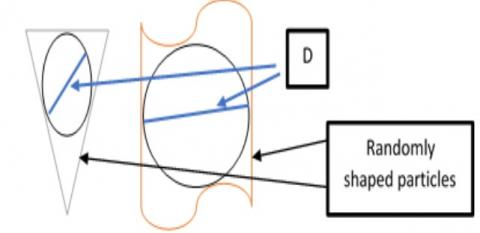Figure 4. Simplification of particle shapes

(1) Thus, the detailed shape of the particles is less important . As a result, the particles in the eddy current control are estimated numerically by approximating their shapes to cylindrical shapes, spheres and disks.

(2) In the present study, we used the shape of 3 mm radius discs and the particle thickness is 1 mm, 1.5 mm, and 2 mm. Also, we chose six waste metals: copper, aluminum, zinc, bronze, lead, and tin, under an excitation frequency of 100 KHz estimated as working frequency. Figure 5 presents the geometry of the control sensor which consists of 580 turns arranged in series, has a height of 13.5 mm, and the inner and outer radius are successively 4.65 mm and 5.6 mm. The distance between the sensor and the load is the lift-off which takes the values: 0.1 mm, 0.88 mm, and 1 mm.

The method is to measure the sensor response by varying the value of the particle thickness and the gap value for each material.

## 5.jpg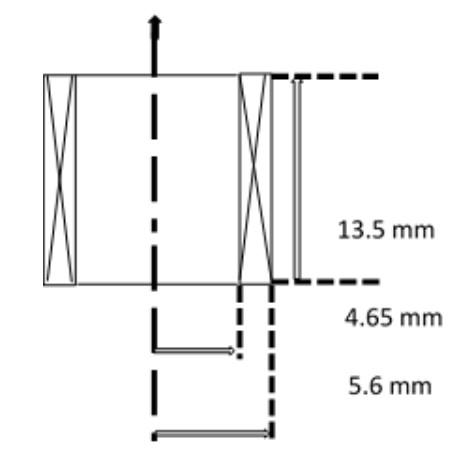Figure 5. Schematic illustration and dimensions of the electromagnetic sensor

4. Results and Discussions

4.1 Effect of the liftoff on the CF signal

The excitation frequency being 100 kHz, Figure 6 shows the module, resistance, and reactance of the sensor impedance for different liftoff values: 0.1 mm, 0.88 mm and 1 mm, and depending on the electrical conductivity of the material. We notice the decrease of the impedance module when the sensor-target distance increases.

The figures clearly show that the reactance is much more sensitive than the liftoff variation resistance. We can consider the reactance of the coil as a source of information to evaluate the liftoff or gap between two plates. The sensitivity is better for small values of the between iron.

## 6.pngFigure 6. Module, resistance, and reactance of the impedance according to the liftoff and the electrical conductivity of the metal waste under 100 KHz

4.2 Effect of particle thickness on the CF signal

We present on the Figure 7 the response of the sensor (Impedance module) for the three values of the thickness depending on the thickness of the particles, for three values of the thickness and depending on the electrical conductivity of the materials.

It can be seen that the modulus of impedance increases with the value evolution of the particle thickness of the samples. We can explain this by the fact that the amplitude of the sensor signal depends on the probed volume, the sensor is sensitive to these variations. The impedance module is more important for high thicknesses. So, this leads to a decrease in the electrical conductivity of the materials.

## 7.png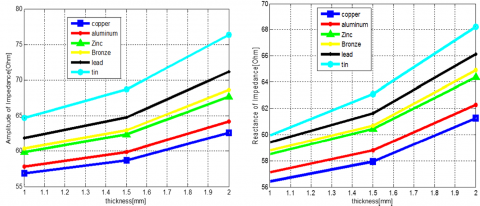Figure 7. Module, resistance, and reactance of the impedance according to the liftoff and the electrical conductivity of the metal waste under 100 KHz

4.3 Characterization of materials depending on particle thickness

The electrical conductivity (Ms / m) can be carried out from a single relation: the impedance depending on the electrical conductivity previously characterized at a given frequency f, appropriately chosen, that is to say in the range where the measurements are sufficiently sensitive . Figure 8 shows the modulus of the impedance depending on the electrical conductivities of the six metal samples with the values of the particle thickness, under an excitation frequency of 100 KHz, and the value of the lift off is set to 0.1 mm.

## 8.jpg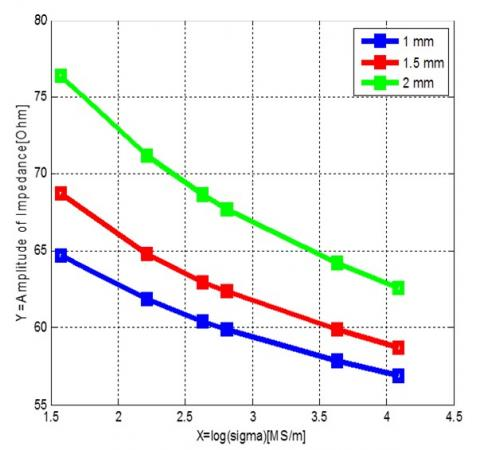Figure 8. The nonlinear regression lines of the impedance module depending on the electrical conductivity with the particle thickness

We note that the CF signal strongly depends on the thickness of the particles, the modulus of the impedance increases depending on the evolution of the thickness of the particles.

Table 1. Characterization of a copper sample

 Thickness [mm] The regression line R Square Conductivity of the copper sample [MS/m] Errors 1 Y=-3*ln$\sigma$+ 69 0.9747 57.1264 2.4736 1.5 Y=-3.8 *ln$\sigma$+ 74 0.9624 56.5117 3.0883 2 Y=-5.33 * ln$\sigma$+ 83.5 0.9685 50.7039 8.8961

Table 1 presents for each value of the particle thickness and estimating the liftoff at 0.1 mm. The linear correlation coefficient between the values of the impedance modules and the values of the conductivity of the samples (R Square), the nonlinear regression line, and the determination of the conductivity of a copper sample by inversion of the direct model of regression, as well as the absolute error.

The accuracy of the estimate can be quantified by the relative error in estimating the electrical conductivity of a copper sample. The estimate of the conductivity for the value of the thickness is equal to 1 mm is correct since the error does not exceed 2.4736%. For the other two values, the estimate is less accurate.

Then, the test of the proposed estimation algorithm gives satisfactory results when the value of the thickness and the value of the lift off are respectively 1mm, and 0.1 mm. The regression line depends on the thickness of the particles. We can conclude that a standard particle thickness must be determined to optimize characterization.

5. Conclusions

The impedance variation is computed as a function of the electric conductivity of the particles Quantitatively, Figure 3 show a good behaviour of the magnetic flux density. The results show the decrease of the impedance module when the sensor-target distance increases. A low sensitivity of control is obtained. It is found that the impedance module is more important for the thicker layers. So, this leads to a decrease in the electrical conductivity of the materials and the characterization of a sample is less accurate. After optimization and sensitivity study, the geometries selected for the value of the lift off and the thickness of the particles are respectively 0.1 mm and 1 mm. To have an optimal characterization, it is necessary to choose a value of the lift off which ensures a good sensitivity to the control sensor, and to determine a standard thickness of the particles to optimize the characterization.

References

 Mercier, J.P., Zambelli, G., Kurz, W. (2002). Introduction to Materials Science. Polytechnic presses and French-speaking universities. ISBN: 2-84299-286-5

 Jung, C.G. (2013). Solid waste treatment: Material and energy recovery. Scientific bulletin of the National Institute for the Environment and Nature Conservation, 12: 50-54.

 Chen, W.K. (1993). Linear networks and systems. algorithms and computer-aided implementations. Advanced In Electrical and Computer EngineeringWadsworth, Belmont, 123-135. https://doi.org/10.1142/0676

 Dwivedia, S.K., Vishwakarmab, M., Soni, A. (2018). Advances and researches on non-destructive testing: A review. Materials Today: Proceedings, 5(2): 3690-3698. https://doi.org/10.1016/j.matpr.2017.11.620

 Kumar, S., Mahto, D. (2013). Recent trends in industrial and other engineering applications of non destructive testing: A review. International Journal of Scientific & Engineering Research, 13(3): 183-195.

 Ghlam, K., Ayad, A., Nafaa, D. (2018). Identification of the metal waste using an electromagnetic sensor. Modelling, Measurement and Control C, 79(2): 17-23. https://doi.org/10.18280/mmc_c.790201

 Kadri, M., Sahli, A., Sahli, S. (2019). Analysis of cylindrical shells by the least squares method. Mathematical Modelling of Engineering Problems, 6(1): 61-68. https://doi.org/10.18280/mmep.060108.

 Santandrea, L., Le Bihan, Y. (2010). Using COMSOL multiphysics in an eddy current non-destructive testing context. Excerpt from the Proceedings of the COMSOL Conference, Paris, France.

 Chifaa, A., Azzedine, H., Elchikh, M., Lebey, T. (2016). Development of A 3d model eddy current testing. International Journal of Engineering Science Invention, 5: 73-81.

 Sohaili, J., Muniyandi, S.K., Mohamad, S.S. (2012). A review on printed circuit board recycling technology. Journal of Emerging Trends in Engineering and Applied Sciences (JETEAS), 3(1): 12-18.

 Mesina, M.B., de Jong, T.P., Dalmijn, W.L. (2003). Improvements in separation of non-ferrous scrap metals using an electromagnetic sensor. Physical Separation in Science and Engineering, 12(1): 87-101. https://doi.org/10.1080/1478647031000139079

 Maraspina, F., Bevilacquaa, P., Remb, P.C. (2004). Modelling the throw of metals and nonmetals in eddy current separations. International Journal of Mineral Processing, 73(1), 1-11. https://doi.org/10.1016/S0301-7516(03)00081-4